## Ncert solutions for class 8 maths chapter 1,Rational Numbers solutions/class viii Rational numbers solutions.

NCERT SOLUTIONS FOR CLASS 8 MATHS CHAPTER 1 RATIONAL NUMBERS Rational Numbers chapter 1 class 8 Maths Ncert solutions are given. First you study the textbook lesson. Observe the example problems and solutions given in the textbook. NCERT class 8 maths solutions  VIII CLASS RATIONAL NUMBERS SOLUTIONS class 8 NCERT  You can see ncert solutions …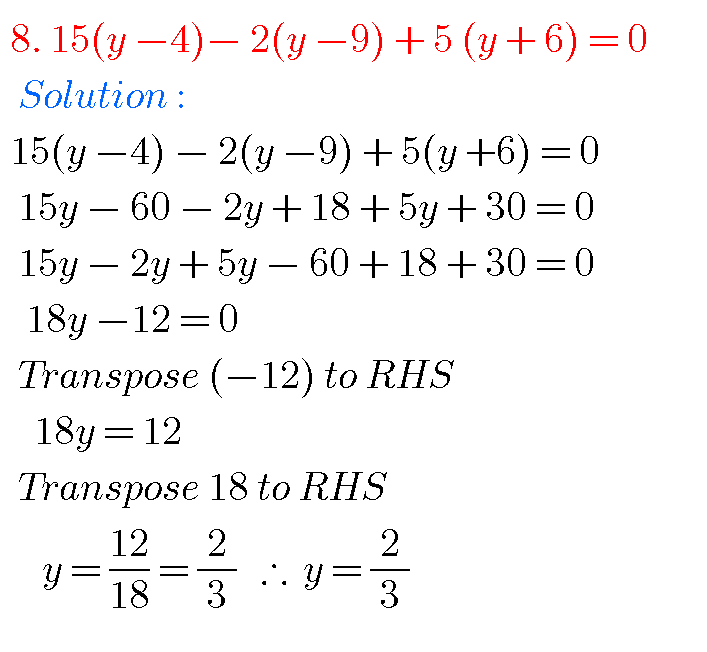## Ncert solutions for class 8 maths chapter 2,Linear Equations in One Variable solutions

NCERT SOLUTIONS FOR CLASS 8  MATHS CHAPTER 2 Class 8 Chapter 2 Linear Equations in One Variable Solutions Maths Ncert are given. First you study the textbook lesson Linear Equations in One Variable very well. You should observe and practice the example problems and solutions given in the textbook. Observe the given below solutions and …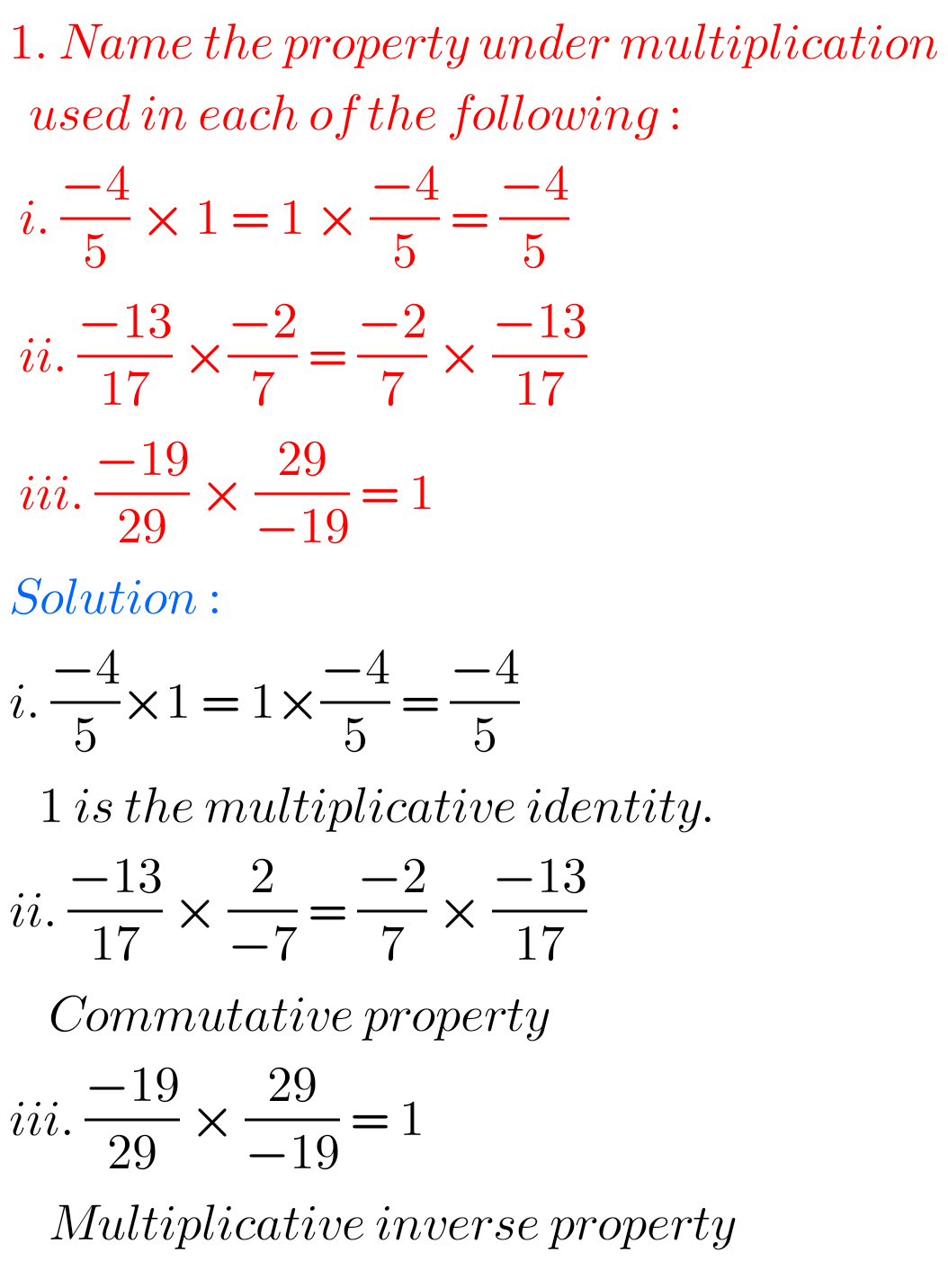## NCERT solutions maths class 8

Class 8 maths solutions NCERT NCERT mathematics class 8 textbook solutions are given for some chapters. You should study the textbook lesson very well. Solutions for Class 8 maths NCERT 1. Rational Numbers 2. Linear Equations in one Variable 3. Understanding Quadrilaterals 4. 5. Squares and Square Roots Maths solutions for class 8 NCERT M Note : Observe the …

## NCERT Maths Class 7 solutions

Maths class 7th NCERT Solutions NCERT mathematics Class 7th solutions for some chapters are given. You should study the textbook lessons very well. You should observe and practice all example problems and solutions are given in the textbook. Observe the given solutions and try them in your own method. Solutions for class 7 NCERT Mathematics …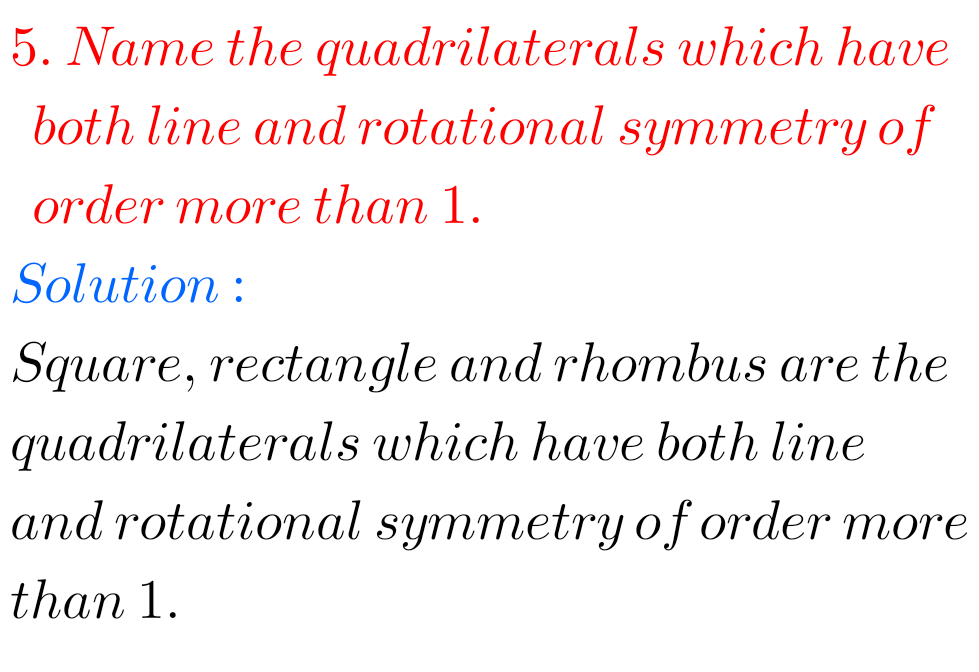## Symmetry Exercise 12.3 class 7 maths NCERT solutions

Symmetry exercise 12.3 solutions class 7 NCERT NCERT class 7 mathematics chapter 12 Symmetry Exercise 12.3 solutions are given. You should practice all problems and solutions are given in the textbook. Observe the given solutions and try them in your own method. You can see. NCERT maths class 7 solutions Symmetry Exercise 12.1 Exercise 12.2 …## NCERT Maths Solutions class 7 Exercise 12.2 Symmetry

Symmetry exercise 12.2 solutions class 7 NCERT NCERT class 7 mathematics chapter 12 Symmetry Exercise 12.2 solutions are given. You should practice all problems and solutions are given in the textbook. Observe the given solutions and try them in your own method. You can see. NCERT maths class 7 solutions Symmetry Exercise 12.1 Exercise 12.2 …## NCERT solutions class 7 maths chapter 12 Symmetry Exercise 12.1

Symmetry exercise 12.1 solutions class 7 NCERT NCERT class 7 mathematics chapter 12 Symmetry Exercise 12.1 solutions are given. You should practice all problems and solutions are given in the textbook. Observe the given solutions and try them in your own method. You can see. NCERT maths class 7 solutions Symmetry Exercise 12.1 Exercise 12.2 …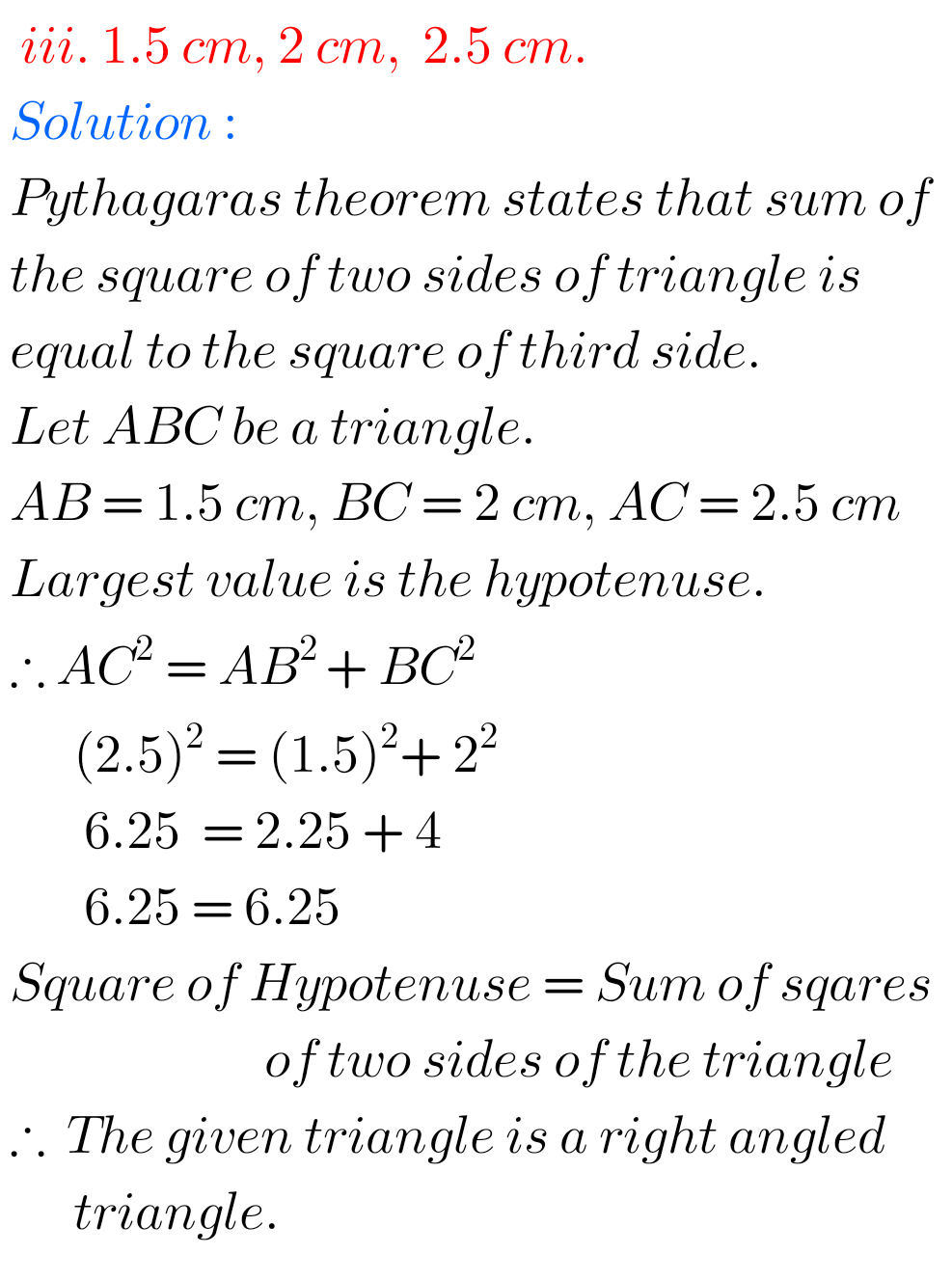## Chapter 6 Exercise 6.5 NCERT maths class 7 The Triangle and its Properties

The Triangle and its Properties Exercise 6.5 solutions NCERT class 7 NCERT mathematics class 7 The Triangle and its Properties Exercise 6.1 solutions are given. First you should study the textbook lesson The Triangle and its Properties very well. You should practice all example problems and solutions are given in the textbook. Observe the given …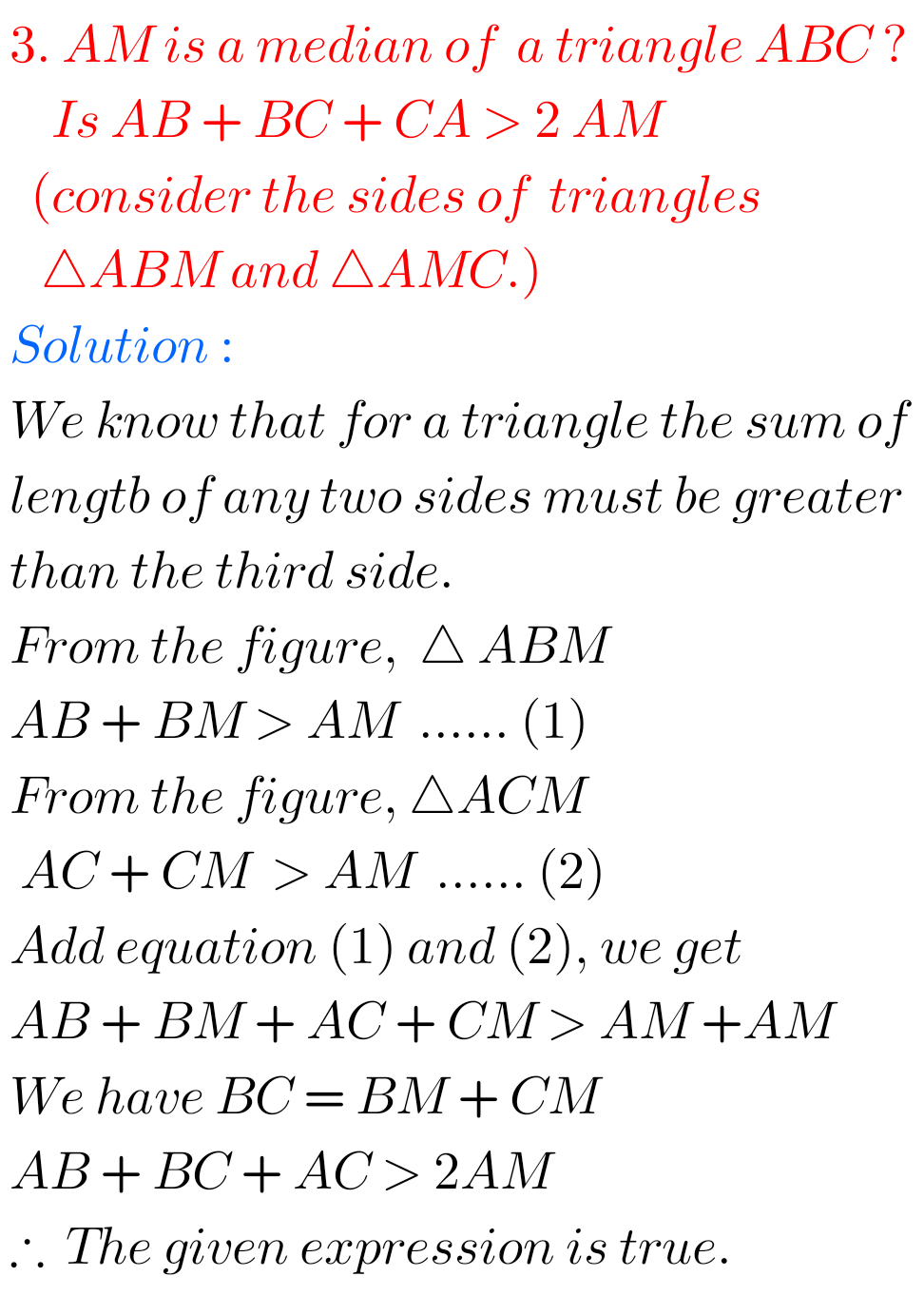## Exercise 6.4 solutions class 7 maths NCERT The Triangle and its Properties

The Triangle and its Properties Exercise 6.4 solutions NCERT class 7 NCERT mathematics class 7 The Triangle and its Properties Exercise 6.4 solutions are given. First you should study the textbook lesson The Triangle and its Properties very well. You should practice all example problems and solutions are given in the textbook. Observe the given …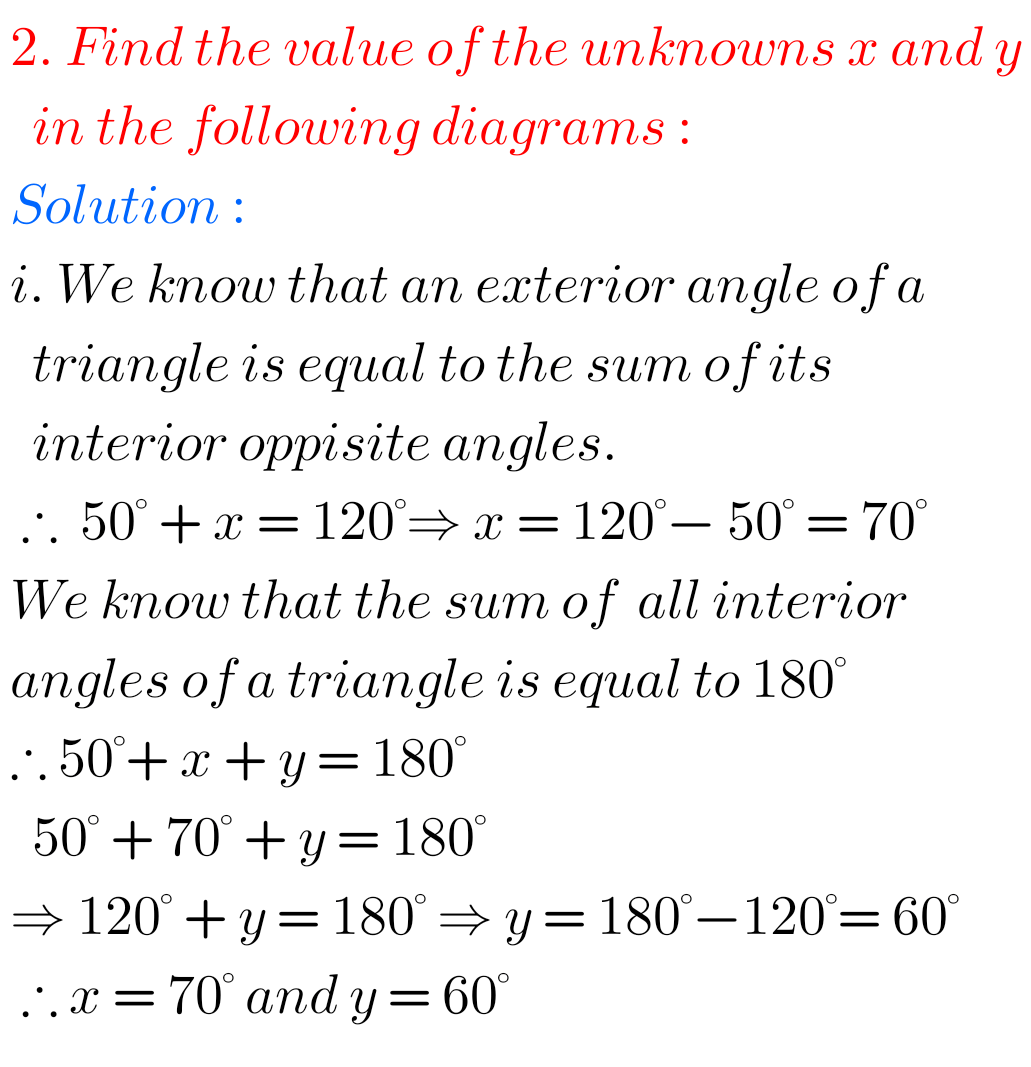## NCERT maths solutions class 7 Exercise 6.3 The Triangle and ita Properties

The Triangle and its Properties Exercise 6.3 solutions NCERT class 7 NCERT mathematics class 7 The Triangle and its Properties Exercise 6.3 solutions are given. First you should study the textbook lesson The Triangle and its Properties very well. You should practice all example problems and solutions are given in the textbook. Observe the given …